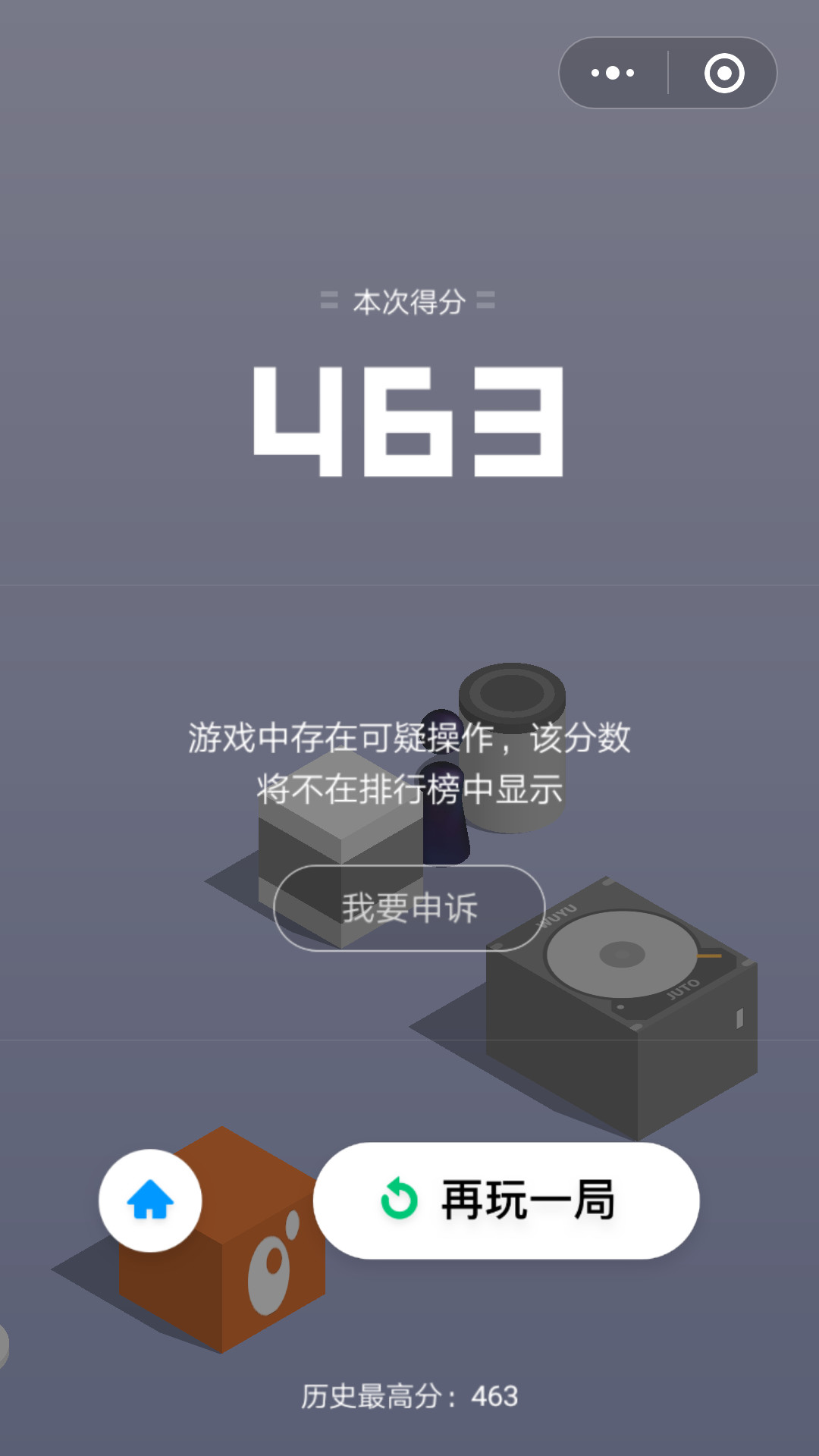### 用Python实现跳一跳自动跳跃

1.  OpenCV：模板匹配。   获得小跳棋中心位置

2.  OpenCV：边缘检测。   获得下一方块中心位置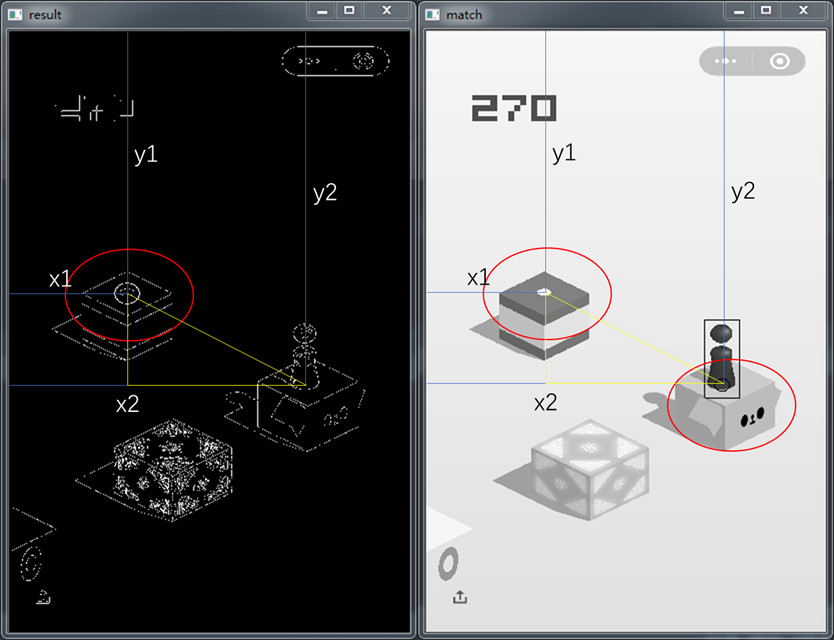`` ``

def get_screenshot():
# 截取手机的屏幕
# 把模拟器里面的文件或文件夹传到电脑上

def jump(distance):
# 设置按压时间,系数为1.35
press_time = int(distance * 1.35)

# 生成随机手机屏幕模拟触摸点,防止成绩无效
# 生成随机整数(0-9),最终数值为(0-90)
rand = random.randint(0, 9) * 10

cmd = (‘adb shell input swipe %i %i %i %i ‘ + str(press_time)) % (320 + rand, 410 + rand, 320 + rand, 410 + rand)

print(cmd)

os.system(cmd)

/ 02 / 跳动实现

`` ``

# 游戏结束的模板图像

def game_over(img):
“””
模板匹配,检测是否要将程序结束
“””
# 如果在游戏截图中匹配到带”再玩一局”字样的模板，则循环中止
res_end = cv2.matchTemplate(img, temp_end, cv2.TM_CCOEFF_NORMED)
if cv2.minMaxLoc(res_end) > 0.95:
print(‘Game over!’)
return True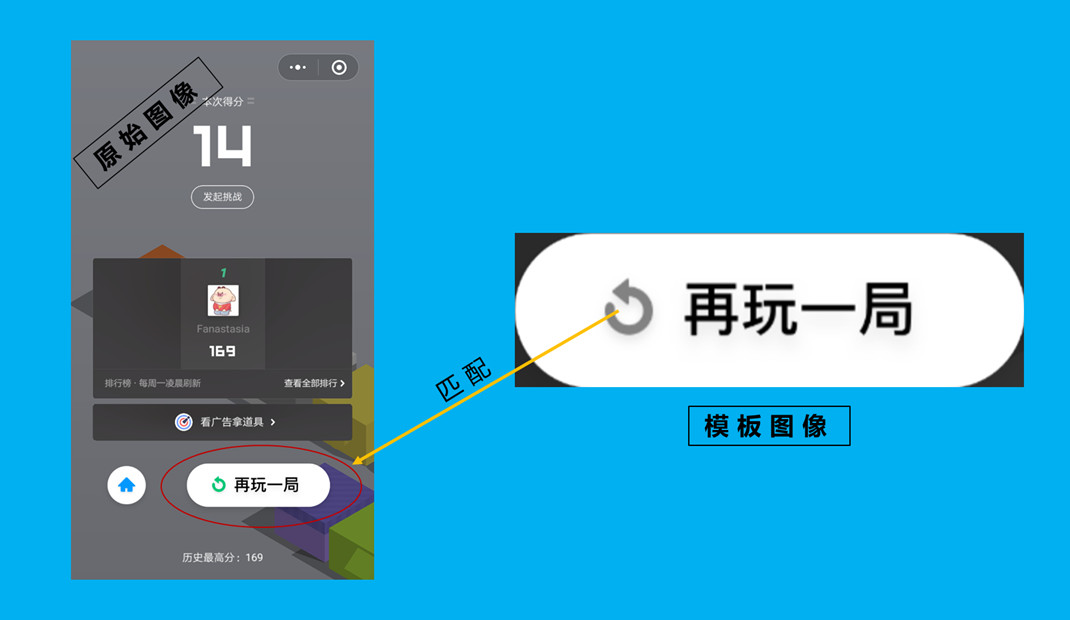`` ``

# 读取小跳棋模板图像
# 获取小跳棋模板图像的高和宽
th, tw = temple.shape[:2]

def get_start(img):
“””
模板匹配,获取跳一跳起点的位置参数(小跳棋)
“””
# 使用标准相关系数匹配,1表示完美匹配,-1表示糟糕的匹配,0表示没有任何相关性
result = cv2.matchTemplate(img, temple, cv2.TM_CCOEFF_NORMED)
# 使用函数minMaxLoc,确定匹配结果矩阵的最大值和最小值(val)，以及它们的位置(loc)
min_val, max_val, min_loc, max_loc = cv2.minMaxLoc(result)
# 得到小跳棋的中心位置参数
return max_loc + 47, max_loc + 208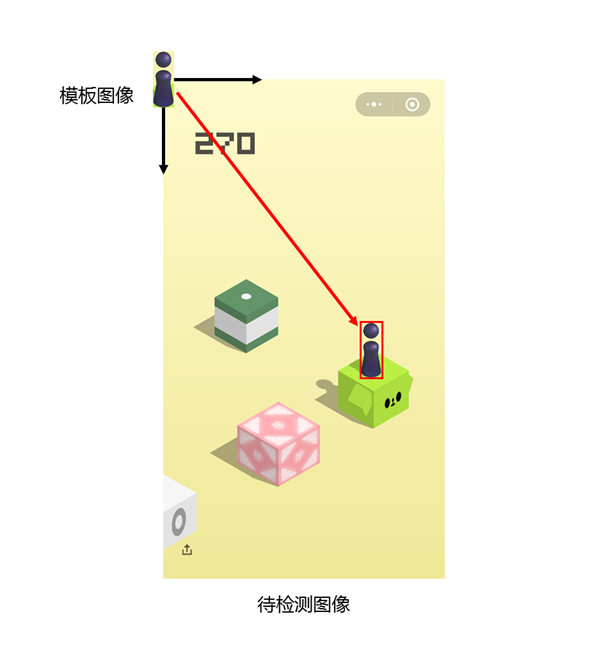`` ``

def get_end(img):
“””
边缘检测,获取跳一跳终点的位置参数(方块)
“””
# 高斯模糊
img_rgb = cv2.GaussianBlur(img, (5, 5), 0)
# 边缘检测
canny_img = cv2.Canny(img_rgb, 1, 10)
# 获得边缘检测图像的高和宽
H, W = canny_img.shape

# 第一个顶点的高度
y_top = np.nonzero([max(row) for row in canny_img[400:]]) + 400
# 第一个顶点的宽度
x_top = int(np.mean(np.nonzero(canny_img[y_top])))

# 跳过小白圈,然后遍历
y_bottom = y_top + 80
for row in range(y_bottom, H):
if canny_img[row, x_top] != 0:
y_bottom = row
break

# 得到方块的中心点
x_center, y_center = x_top, (y_top + y_bottom) // 2
return x_center, y_center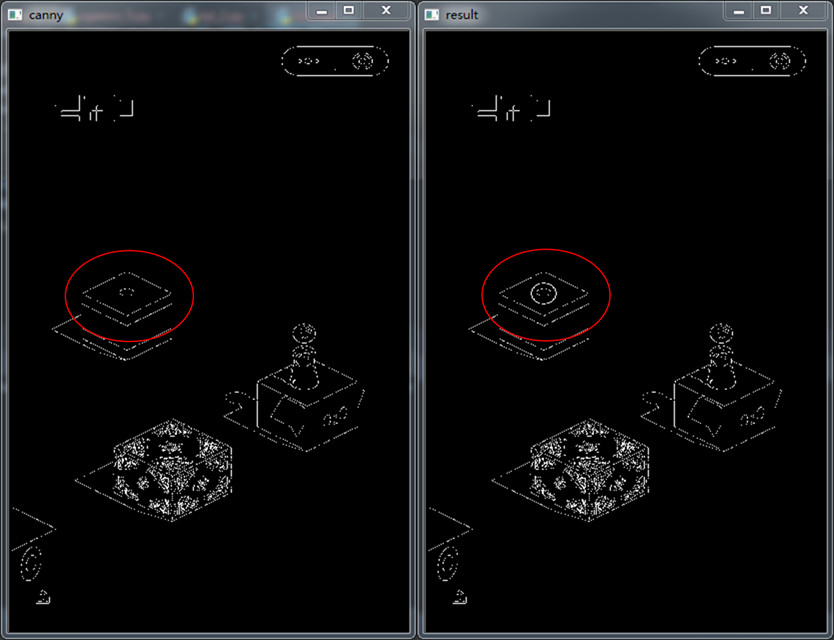`` ``

# 循环直到游戏失败结束
for i in range(10000):
# 将安卓手机上的截图移到电脑当前文件夹下
get_screenshot()
# 读取截图图像

# 游戏结束
if game_over(img):
break

# 得到起点位置参数
x_start, y_start = get_start(img)
# 获取终点位置参数
x_end, y_end = get_end(img)

# 将起点位置绘制出来,一个圆
cv2.circle(img, (x_start, y_start), 10, 255, -1)
# 将终点位置绘制出来,一个圆
img_end = cv2.circle(img, (x_end, y_end), 10, 255, -1)
# 保存图片
cv2.imwrite(‘end.png’, img_end)

# 计算起点和终点的直线距离,勾三股四弦五
distance = (x_start – x_end) ** 2 + (y_start – y_end) ** 2
distance = distance ** 0.5

# 根据获得的距离来设置按压时长
jump(distance)
time.sleep(1.3)

https://v.qq.com/x/page/e0887dhjvux.html

/ 03 / 总结